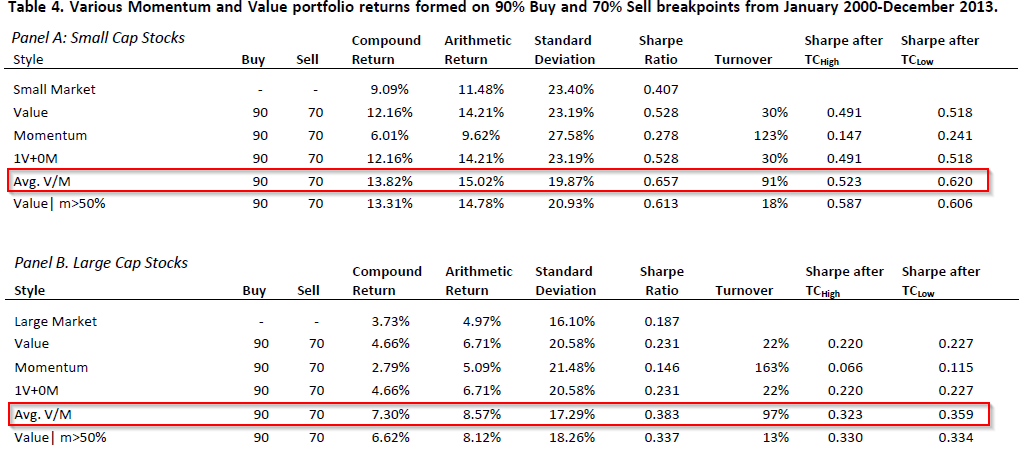### Abstract:

This paper considers several popular portfolio implementation techniques that maximize exposure to value and/or momentum stocks while taking into account transaction costs. Our analysis of long-only strategies illustrates how a strategy that simultaneously incorporates both value and momentum outperforms a strategy that combines pure-play value and momentum portfolios that are formed independently. There are two advantages of the simultaneous strategy. The first is the reduction in transaction costs; the second is better utilization of unfavorable value and momentum information in a long-only portfolio. Our analysis also addresses the optimal way to combine the faster-moving momentum signal with the slower-moving value signal.

### Alpha Highlight:

Numerous academic papers examine pure-play value and momentum portfolios. Sheridan Titman–one of the authors on this paper–has a well-established and respected group of papers on momentum. Why he decided to join in on this paper is a bit puzzling, given the paper’s weak robustness analysis and “practitioner-focused” bent. Nonetheless, the paper asks an interesting question: “Why not combine value and momentum in one portfolio and “kill two birds with one stone?” This paper is not the first to consider this issue. Asness, Moskowitz and Pedersen (2012) shows that even 50/50 equal combination of value and momentum can significantly outperform pure-play strategies. Asness (1997) and Daniel & Titman (1999) show that momentum and value are negatively correlated. Stivers and Sun (2009) use Return Dispersion (RD) as a proxy for volatility and thus generate a timing signal to combine these two strategies dynamically. The point of all this research is clear: momentum is cool; value is cool; combining the 2 is awesome. The authors of this paper use two simple methods to combine value and momentum and find the following benefits relative to “pure-play” value or momentum strategies:

• Reductions in transaction costs
• Higher Sharpe Ratios

The authors highlight that, “Momentum is a relatively fast moving characteristic, since returns from year to year are relatively independent, while value is a relatively slow-moving characteristic, since it is based on levels rather than changes in market values.” Thus, combining the fast and slow characteristics can better utilize the unfavorable information embedded in the characteristics. The two simple combination strategies are:

1. Strategy 1 — Average V/M strategy: Ranks firms by Momentum and Value separately, and then compute the average rank (Rj), to calculate the stock’s Average V/M score.
• The Avg. V/M score has exposure to both signals equally. The disadvantage of this simple strategy is that one signal has the ability to out-weigh the other. Thus, the changes in momentum scores have a large influence on trading, which lead to higher trading costs.
2. Strategy 2 — Value|m>X: Rather than selecting stocks based on a combined signal, after initial positions are chosen, trades are initiated only when both value and momentum signals are sufficiently favorable.
• This strategy has greater value exposure and less momentum exposure.

Which of the two combination strategies works better? Below is a main result table of the paper.  The table shows the performance of 5 different strategies from 2000 to 2013 (we focus on 4): Value only, Momentum only, Average V/M strategy, and Value|m>50% strategy.The results are hypothetical results and are NOT an indicator of future results and do NOT represent returns that any investor actually attained. Indexes are unmanaged, do not reflect management or trading fees, and one cannot invest directly in an index. Additional information regarding the construction of these results is available upon request.

### Key Findings:

1. From the table above, both the two combination strategies work well. They generate higher returns, lower standard deviations, and higher Sharpe Ratios than the Value or Momentum strategy only.
2. The Avg. V/M strategy performs relatively better (highest Sharpe ratios for both large and small capitalization stocks) in this paper.

These two methods are easy to design and apply. But the data period in this paper is short, thus making the results not very informative or unique.